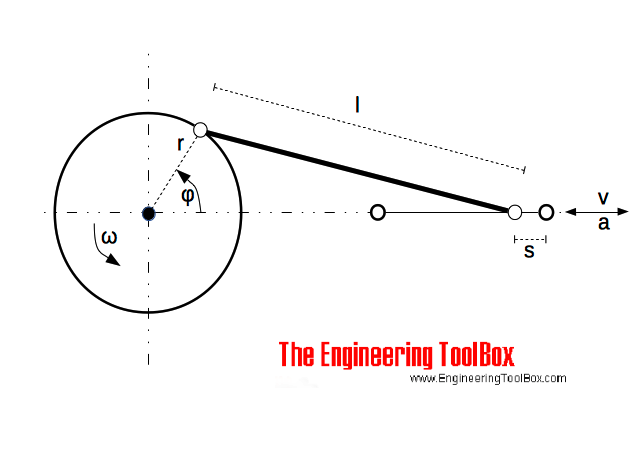Engineering ToolBox - Resources, Tools and Basic Information for Engineering and Design of Technical Applications!

# Conn-Rod Mechanism

## The connecting rod mechanism.A connecting rod - conn-rod - mechanism converts rotating motion to reciprocating motion - or vice versa.

The position of the rod can be expressed as

s = r (1 - cos φ) + (λ / 2) r sin2φ                                (1)

where

s = position of the rod (m)

r = radius of crank (m)

φ = ω t

= 2 π ns t

= angular position of crank (rad)

ω = crank angular velocity (rad/s)

t = time (s)

ns = revolution per second (1/s)

λ = r / l

= crank ratio

l = length of rod (m)

The velocity of the rod can be expressed as

v = ω r sin φ (1 + λ cos φ)                               (2)

where

v = velocity of rod (m/s)

The acceleration of the rod can be expressed as

a = ω2 r (cos φ + λ cos 2φ)                                (3)

where

a = acceleration of rod (m/s2)

## Related Topics

• ### Mechanics

Forces, acceleration, displacement, vectors, motion, momentum, energy of objects and more.

## Related Documents

• ### Acceleration

Change in velocity vs. time used.
• ### Angular Motion - Power and Torque

Angular velocity and acceleration vs. power and torque.
• ### Belt Transmissions - Speed and Length of Belts

Calculate length and speed of belt and belt gearing.
• ### Centripetal and Centrifugal Acceleration Force

Forces due to circular motion and centripetal / centrifugal acceleration.
• ### Flywheels - Kinetic Energy

The kinetic energy stored in flywheels - the moment of inertia.
• ### Formulas of Motion - Linear and Circular

Linear and angular (rotation) acceleration, velocity, speed and distance.
• ### Gear Reducing Formulas

Output torque, speed and horsepower with gears.
• ### Gears

Gears effort force vs. load force.
• ### Mass Moment of Inertia

The Mass Moment of Inertia vs. mass of object, it's shape and relative point of rotation - the Radius of Gyration.
• ### Movement Ratio

Machines and movement ratio (velocity ratio).
• ### Torque - Work done and Power Transmitted

The work done and power transmitted by a constant torque.

## Engineering ToolBox - SketchUp Extension - Online 3D modeling!

Add standard and customized parametric components - like flange beams, lumbers, piping, stairs and more - to your Sketchup model with the Engineering ToolBox - SketchUp Extension - enabled for use with older versions of the amazing SketchUp Make and the newer "up to date" SketchUp Pro . Add the Engineering ToolBox extension to your SketchUp Make/Pro from the Extension Warehouse !

We don't collect information from our users. More about

## Citation

• The Engineering ToolBox (2016). Conn-Rod Mechanism. [online] Available at: https://www.engineeringtoolbox.com/connecting-rod-mechanism-d_1936.html [Accessed Day Month Year].

Modify the access date according your visit.

9.29.12